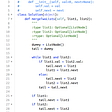# Part 1: Introduction

In order to represent data in Python, we have the following options:

• write a class that organize data
• use named tuple / dictionary or their enhanced versions (from typing)
• employ dataclass

We recommend to use dataclass as it provides a lot of possibilities.

However, using dataclass can be problematic from the software engineering perspective:

These are classes that have fields, getting and setting methods for fields, and nothing else.Such classes are dumb data holders and are often being manipulated in far too much detail by other classes.

— from Refactoring of Martin Fowler and Kent Beck

The main idea of OOP is to place behavior and data together in the same code unit.

# Part 2: NamedTuple/NamedDict

namedtuple

`from collections import namedtupleCoordinate = namedtuple('Coordinate', 'lat long')`

namedtuple from typing

`from typing import NamedTupleclass Coordinate(NamedTuple):    lat: float    long: float    def __str__(self):        ns = 'N' if self.lat >= 0 else 'S'        we = 'E' if self.long >= 0 else 'W'        return f'{abs(self.lat):.1f}°{ns}, {abs(self.long):.1f}°{we}'a = Coordinate(3.4, 5.6)`

Example can be found in my github.

# Part 3: Dataclasses

`from dataclasses import dataclass@dataclass(frozen=True)class Coordinate:    lat: float    long: float=3.4    def __str__(self):        ns = 'N' if self.lat >= 0 else 'S'        we = 'E' if self.long >= 0 else 'W'        return f'{abs(self.lat):.1f}°{ns}, {abs(self.long):.1f}°{we}'`

(1) The default setting in @dataclass is as follows:

`@dataclass(*, init=True, repr=True, eq=True, order=False,              unsafe_hash=False, frozen=False)`

This default setting can be modified for each class member:

`from dataclasses import dataclass, field@dataclass(order=True)class PlayingCard:   sort_index: int = field(init=False, repr=False)   val:int    def __post_init__(self):         self.sort_index = self.val*2    a= PlayingCard(3)print(a)print(a.sort_index)`

(2) Field options do not include mutable types, and this is because mutable default values are a common source of bugs for Python developers. Therefore, @dataclass does not support mutable type initialization. However, it allows to define class member variable as mutable.

`@dataclassclass MyNumber:    a:list obj = MyNumber(['a','b'])print(obj)`

However, the following definition is problematic

`@dataclassclass MyNumber:    a:list=['a','b'] obj = MyNumber()  # error will occur`

In order to solve this problem, a work-around solution is to use `field` method:

Example 1: empty list

`from dataclasses import dataclass, field@dataclassclass ClubMember:    name: str    guests: list = field(default_factory=list)        a= ClubMember("my")a.guests.append('3')a.guests.append('4')print(a)`

Example 2: list with initialization

`import randomfrom typing import Listdef get_random_marks():    return [random.randint(1,10) for _ in range(5)]@dataclassclass Student:    marks: list = field(default_factory=get_random_marks)a = Student()print(a)`

Example 3: list with type annotation and initialization

`from dataclasses import fieldfrom typing import Listdef get_random_marks():    return [random.randint(1,10) for _ in range(5)]@dataclassclass Student:    marks: List[int] = field(default_factory=get_random_marks)        b = Student()print(b)`

(3) class attribute vs class member

`@dataclassclass MyClass:    all_set_as_class_attribute = set({'p1','p2'})    all_set:seta = MyClass({'a','b'})b = MyClass({'aa','bb'})print(a)print(b)print(a.all_set_as_class_attribute)a.all_set_as_class_attribute.add('ppppppp')print(b.all_set_as_class_attribute)print(MyClass.all_set_as_class_attribute)`

Its output is as follows:

`MyClass(all_set={'a', 'b'})MyClass(all_set={'aa', 'bb'}){'p1', 'p2'}{'p1', 'p2', 'ppppppp'}{'p1', 'p2', 'ppppppp'}`

My understanding is that when the variable in the class is mutable, then this variable can be shared with many other class objects.

My understanding is that when the variable is initialized from the beginning, it will be regarded as class variable.

`@dataclassclass MyClass:         obj_name:str='ab'a = MyClass()print(a)b = MyClass('ef')print(b)print(MyClass.obj_name)`

Its output is:

`MyClass(obj_name='ab')MyClass(obj_name='ef')ab`

(4)` __post_init__` is used to post-process the initialized @dataclass object.

`@dataclassclass MyClass:    all_objects = set() # all_objects:ClassVar[Set[str]] = set()    obj_name:str='a'        def __post_init__(self):        cls = self.__class__        if self.obj_name:           cls.all_objects.add(self.obj_name) a = MyClass('a')b = MyClass('b')c = MyClass('c')d = MyClass('b')print(a.all_objects)`

Its output is:

`{'a', 'b', 'c'}`

Another very good example of `__post_init__` provides a way of looking up an item from the database

`from dataclasses import dataclass, InitVar @dataclassclass C:        i: int        j: int = None        database: InitVar[int] = None        def __post_init__(self, database):                if self.j is None and database is not None:                        self.j = database.lookup('j')c = C(10, database=my_database)`

In this example `InitVar` means that variable must be initialized by the class definition.

My understanding of of `InitVar` and `field(init=False, repr=False)` is that InitVar is not regarded as class member variable.

InitVar Example

`from dataclasses import dataclass, InitVar @dataclassclass C:        i: int        j: int = None        database: InitVar[int] = None        def __post_init__(self, database):                if self.j is None and database is not None:                        self.j = self.i in database#database.lookup('j')c = C(10, database=["a", "b", "c"])print(c)`

field Example

`from dataclasses import dataclass, field@dataclass(order=True)class PlayingCard:   sort_index: int = field(init=False, repr=False)   val:int    val2:int   def __post_init__(self):         self.sort_index = self.val2*2    a= PlayingCard(3,30)print(a)print(a.sort_index)`

# Part 4: Struct

`struct` is used to construct data structure for reading C/C++ structure data.

Structure in C++

`struct MetroArea {    int year;    char name;    char country;    float population;};`

`from struct import unpackFORMAT = 'i12s2sf' def text(field: bytes) -> str:                 # <2>       octets = field.split(b'\0', 1)          # <3>      return octets.decode('cp437')              # <4> with open('metro_areas.bin', 'rb') as fp:      # <5>        data = fp.read() for fields in iter_unpack(FORMAT, data):       # <6>        year, name, country, pop = fields        place = text(name) + ', ' + text(country)  # <7>          print(f'{year}\t{place}\t{pop:,.0f}')`

`struct` and `memoryview` are used to interpret bytes as packed binary data.

# Part 5: Reference

Blogs

Book

Codes

--

--

--

Love podcasts or audiobooks? Learn on the go with our new app.

## Is it possible to build my app with no-code?## Meta-Arguments for Terraform## Using RDS and EC2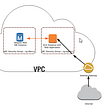## Running mongodb in replica set mode in windows as a service.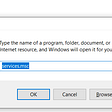## How to choose the needed technology stack for your project?## Setting Up Unity: Part 1 — Unity and VS Code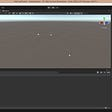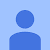## Python Lesson : Lists in Python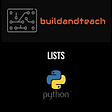## The idiosyncrasy of python linked list